﻿ Some Identities Involving Common Factors of k-Fibonacci and k-Lucas Numbers

Some Identities Involving Common Factors of k-Fibonacci and k-Lucas Numbers

Deepika Jhala, G.P.S. Rathore, Bijendra SinghOPEN ACCESSPEER-REVIEWED

Some Identities Involving Common Factors of k-Fibonacci and k-Lucas Numbers

Deepika Jhala1,, G.P.S. Rathore2, Bijendra Singh1

1School of Studies in Mathematics, Vikram University, Ujjain (India)

2College of Horticulture, Mandsaur (India)

Abstract

Fibonacci sequence stands as a kind of super sequences with fabulous properties. In this paper we present identities involving Common factors of k-Fibonacci and k-Lucas number. Also Binet’s formula will employ to this identity.

• Jhala, Deepika, G.P.S. Rathore, and Bijendra Singh. "Some Identities Involving Common Factors of k-Fibonacci and k-Lucas Numbers." American Journal of Mathematical Analysis 2.3 (2014): 33-35.
• Jhala, D. , Rathore, G. , & Singh, B. (2014). Some Identities Involving Common Factors of k-Fibonacci and k-Lucas Numbers. American Journal of Mathematical Analysis, 2(3), 33-35.
• Jhala, Deepika, G.P.S. Rathore, and Bijendra Singh. "Some Identities Involving Common Factors of k-Fibonacci and k-Lucas Numbers." American Journal of Mathematical Analysis 2, no. 3 (2014): 33-35.

 Import into BibTeX Import into EndNote Import into RefMan Import into RefWorks

1. Introduction

The Fibonacci sequence and the Lucas sequence are the two shining stars in the vast array of integer sequences. They have fascinated both amateurs and professional mathematicians for centuries, and they continue to charm us with their beauty, abundant applications, and ubiquitous habit of occurring in totally surprising and unrelated places.

The Fibonacci numbers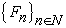are terms of the sequence {0, 1, 1, 2, 3, 5, 8, 13, 21,. ..}, where each term is the sum of the two previous terms, beginning with the values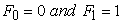. The Lucas numbers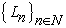are terms of the sequence {2, 1, 3, 4, 7, 11, 18, 29, 47,...}, where each term is the sum of the two previous terms, beginning with the values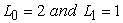. Many kinds of generalizations of Fibonacci numbers and Lucas numbers have been presented in the literature [6, 7, 9]. One example of this recent generalization is k-Fibonacci numbers  and k-Lucas numbers , which are defined by the recurrence relation, depend on one real parameter k. Falcon and Plaza  defined k- Fibonacci hyperbolic functions and deduced some properties of k- Fibonacci hyperbolic functions related with the analogous identities for the k- Fibonacci numbers. Falcon  studied the k- Lucas numbers and proved various properties related with the k- Fibonacci numbers. Thongmoon  and Hoggatt  defined various identities for the Fibonacci and Lucas numbers. Gupta and Panwar , present generalized identities involving common factors of generalized Fibonacci, Jacobsthal and jacobsthal-Lucas numbers and Binet’s formula will employ to obtain the identities. There are a lot of identities of Fibonacci and Lucas numbers described in [1, 8]. In this paper, we present generalized identities involving common factors of k- Fibonacci, and k-Lucas numbers.

2. The K-Fibonacci and the K-Lucas Numbers

Before presenting our main results, we will need to introduce some known results and notations.

The k-Fibonacci numbers have been defined in  for any real k as follows:

For any positive real number k, the k-Fibonacci sequence say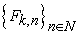is define recurrently by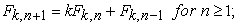(1)

With initial condition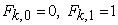(2)

Particular cases of the k-Fibonacci sequence are

If k = 1, the classical Fibonacci sequence is obtained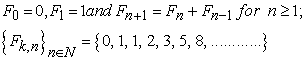If k = 2, the classical Pell sequence is obtained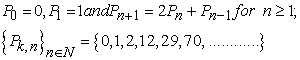If k = 3, the following sequence is obtained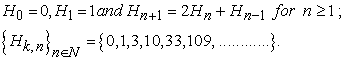Binet formula for the nth k-Fibonacci numbers is given by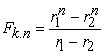(3)

where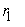,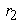are the roots of the characteristic equation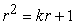and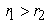;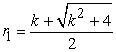,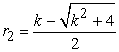which gives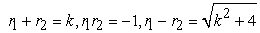(4)

Similarly for any positive real number k, the k-Lucas sequence  say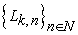is define recurrently by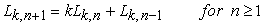(5)

with initial condition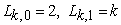(6)

Particular cases of the k-Lucas sequence are

If k = 1, the classical Lucas sequence is obtained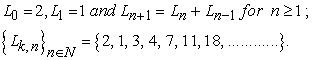If k = 2, the classical Pell- Lucas sequence is obtained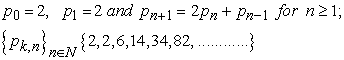If k = 3, the following sequence is obtained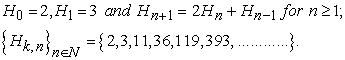Binet formula for the nth k-Lucas numbers is given by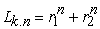(7)

where,are the roots of the characteristic equation which are given in equation (4).

There are a lot of identities for the common factor of Fibonacci and the Lucas numbers define in .

3. Identities for The Common Factors of K-Fibonacci and K-Lucas Number

In this section we present generalized identities involving common factors of k-Fibonacci and k-Lucas numbers. We shall use the Binet’s formula for the k-Fibonacci numbers and k-Lucas numbers for derivation.

3.1. Proposition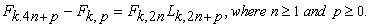Proof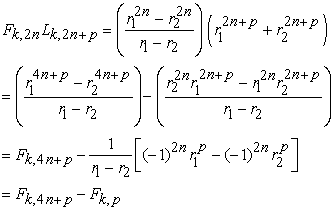(8)
3.2. Proposition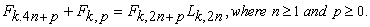Proof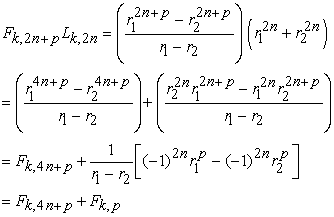(9)
3.3. Proposition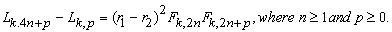Proof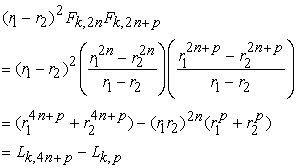(10)
3.4. Proposition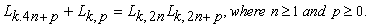Proof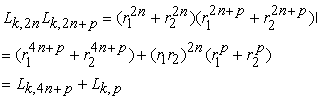(11)
3.5. Proposition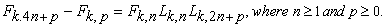Proof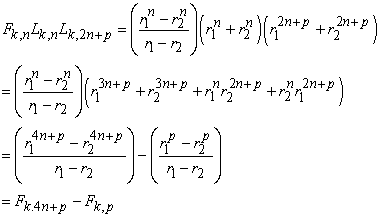(12)
3.6. Proposition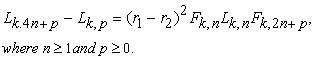Proof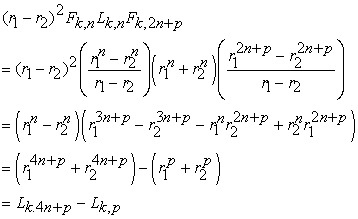(13)
3.7. Proposition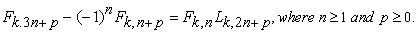Proof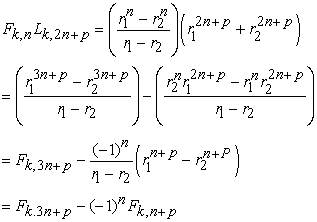(14)

In , if we take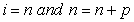in Lemma 1(i), then we have the following proposition.

3.8. Proposition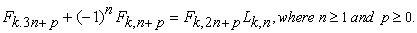Proof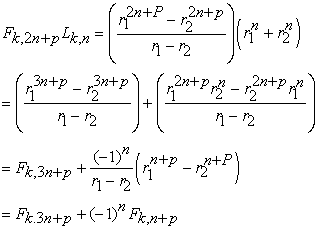(15)
3.9. Proposition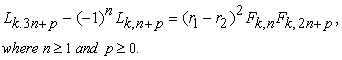Proof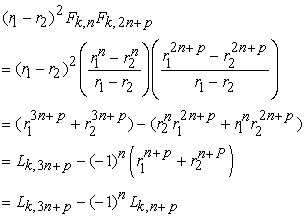(16)

In , if we take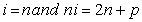in Lemma 1(ii), then we have the following proposition.

3.10. Proposition

Proof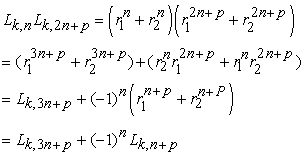(17)

For different values of p, Proposition (3.1) to Proposition (3.10) can be expressed for even and odd k-Fibonacci and k-Lucas numbers.

Identities (8) to (17) give a relation between k-Fibonacci and k-Lucas numbers by using their subscripts.

4. Conclusion

In this paper we present generalized identities involving common factors of k- Fibonacci and k-Lucas numbers. Mainly Binet’s formula employ for the identities. The concept can be executed for generalized second order recursive sequences as well as polynomials.

References

  Benjamin, A. T. and Quinn, J. J., “Recounting Fibonacci and Lucas identies”, The College Mathematics Journal, 30 (5) (1999), 359-366.In article CrossRef  Falcon, S. & Plaza, A., “On the Fibonacci k-numbers”, Chaos, Solitons & Fractals, 32 (5), (2007), 1615-24.In article CrossRef  Falcon S. and Plaza A., The k-Fibonacci hyperbolic functions, Chaos Solitons and Fractals, 38 (2) (2008), 409-420.In article CrossRef  Falcon, S., “On the k-Lucas Numbers”, Int. J. Contemp. Math. Sciences, 6 (21), (2011), 1039-1050.In article  Hoggatt, V.E. Jr., “Fibonacci and Lucas Numbers”, Houghton – Mifflin Co., Boston (1969).In article  Horadam A. F., A generalized Fibonacci sequences, Mathematical Magazine. 68 (1961), 455-459.In article  Horadam A. F. and Shanon A. G.., Generalized Fibonacci triples, American Mathmatical Monthly, 80 (1973), 187-190.In article CrossRef  Koshy, T., “Fibonacci and Lucas Numbers with Applications”, John Wiley, New York (2001).In article CrossRef  Kilic E., The Binet formula, sums and representation of generalized Fibonacci p-numbers, Eur. J. Combin, 29 (3) (2008), 701-711.In article CrossRef  Panwar, Y. K., B. Singh and Gupta, V. K., “identities of Common Factors of generalized Fibonacci, Jacobsthal and Jacobsthal-Lucas numbers”, Applied Mathematics and Physics, 1 (4), (2013), 126-128.In article  Thongmoon, M., “New Identities for the Even and Odd Fibonacci and Lucas Numbers”, Int. J. Contemp. Math. Sciences, 4 (14), (2009), 671-676.In article  Thongmoon, M., “Identities for the common factors of Fibonacci and Lucas numbers”. International Mathematical Forum, 4 (7), (2009), 303-308.In article  Yilmaz, N., Taskara, N., Uslu,K., Yazlik, Y., On the binomial sums of k-Fibonacci and k-Lucas Sequences, American institue of Physics (AIP) Conf. Proc.In article